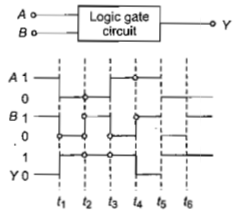The following figure shows a logic gate circuit with two inputs A and B and the output Y. The voltage waveforms of A, B and Y are as givenThe logic gate is

(a) $\mathrm{NOR}$ gate                                                 (b) $\mathrm{OR}$ gate

(c)  gate                                                 (d) gate

Explanation is a part of a Paid Course. To view Explanation Please buy the course.

Difficulty Level: